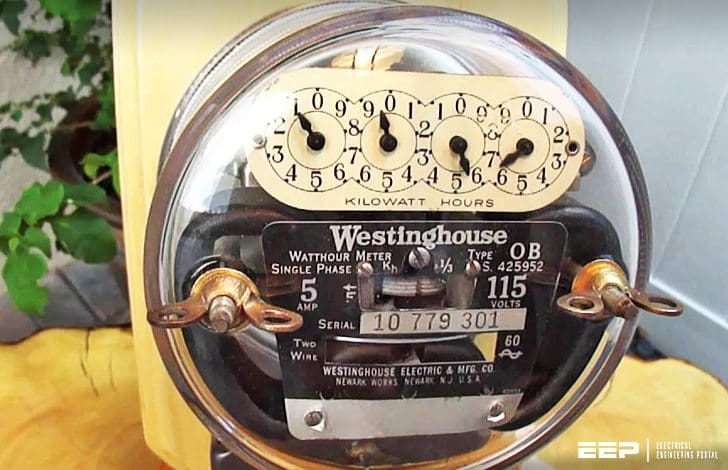Get access to premium HV/MV/LV technical articles, electrical engineering guides and papers. Check our new membership plans and prices!

# The basics of single-phase and three-phase AC circuits for students

Home / Download Center / Electrical Engineering Books and Technical Guides / Electrical engineering guides / The basics of single-phase and three-phase AC circuits for students

## Measurement of power in a 3-phase circuit

In this lesson, the measurement of total power in a three-phase circuit, both balanced and unbalanced, is discussed. The connection diagram for two-wattmeter method, along with the relevant phasor diagram for balanced load, is described.The basics of single-phase and three-phase AC circuits for students (on photo: Westinghouse Vintage Electric Meter Lamp)

After going through this lesson, the students will be able to answer the following questions:

1. How to connect the two-wattmeter to measure the total power in a three-phase circuit – both balanced and unbalanced?
2. Also how to find the power factor for the case of the above balanced load, from the reading of the two-wattmeter, for the two types of connections – star and delta?

### Two-wattmeter method of power measurement

The connection diagram for the measurement of power in a three-phase circuit using two wattmeters, is given in Figure 1. This is irrespective of the circuit connection – star or delta. The circuit may be taken as unbalanced one, balanced type being only a special case.

Please note the connection of the two wattmeters.

The current coils of the wattmeters, 1 & 2, are in series with the two phases, R and B , with the pressure or voltage coils being connected across R − Y and B − Y respectively. Y is the third phase, in which no current coil is connected.

If star-connected circuit is taken as an example, the total instantaneous power consumed in the circuit is:
W =iRN′ × vRN′ + iYN′ ⋅ vYN′ + iBN′ × vBN′

Each of the terms in the above expression is the instantaneous power consumed for the phases. From the connection diagram, the current in, and the voltage across the respective (current, and pressure or voltage) coils in the wattmeter, W1 are iRN′ and vRY = vRN′ − vYN′.

So, the instantaneous power measured by the wattmeter, W1 is:
W1 = iRN’ × vRY = iRN × (vRN′ − vYN′)

Similarly, the instantaneous power measured by the wattmeter, W2 is:
W2 = iBN′ × vBY = iBN′ × (vBN′ − vYN′)

So, it can be concluded that the sum of the two wattmeter readings is the total power consumed in the three-phase circuit, assumed here as a star-connected one. This may also be easily proved for delta-connected circuit.

As no other condition is imposed, the circuit can be taken as an unbalanced one, the balanced type being only a special case, as stated earlier.